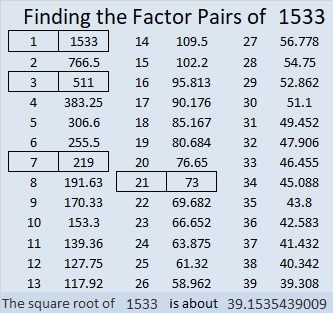# 1533 Double Double Toil and Trouble

Contents

### Today’s Puzzle:

Solving this cauldron-looking mystery level puzzle might be double-double toil and trouble.  Why? There are four sets of clues that have more than one possible common factor. Nevertheless, the puzzle has only one solution, and you can find it if you let logic and a basic multiplication table be your guide.### Factors of 1533:

Double Double Toil and Trouble… You can use two divisibility tricks to find the factors of 1533, and the second trick uses doubling.

Is 1533 divisible by 3? The trick for finding numbers divisible by 3 is to add up their digits. If the sum is divisible by 3, so is the original number:
1 + 5 + 3 + 3 = 12, so 1533 is divisible by 3.
It really is! See what I mean: 1533 ÷ 3 = 511.

Is it divisible by 7? Look at the previous quotient, 511. Separate the last digit from the rest and subtract its double from the first part: 51 – 2 = 49, so 511 is divisible by 7, and its multiple, 1533, is also divisible by 7.

(We could have used the same doubling trick TWICE on 1533, but that definitely would have been double-double, toil, and trouble. Just dividing 1533 by 7 to get 219 would be easier!)

• 1533 is a composite number.
• Prime factorization: 1533 = 3 × 7 × 73.
• 1533 has no exponents greater than 1 in its prime factorization, so √1533 cannot be simplified.
• The exponents in the prime factorization are 1, 1, and 1. Adding one to each exponent and multiplying we get (1 + 1)(1 + 1)(1 + 1) = 2 × 2 × 2 = 8. Therefore 1533 has exactly 8 factors.
• The factors of 1533 are outlined with their factor pair partners in the graphic below.### More Facts About the Number 1533:

1533 is the hypotenuse of a Pythagorean triple, and you can apply those same two divisibility tricks to all three numbers in that triple. (Note: 3 × 7 = 21)
1008-1155-1533 which is 21 times (48-55-73)

1533 is the difference of two squares in FOUR different ways:
767² – 766² = 1533,
257² – 254² = 1533,
113² – 106² = 1533, and
47² – 26² = 1533.

This site uses Akismet to reduce spam. Learn how your comment data is processed.